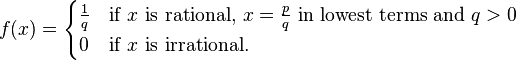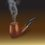# The continuity of Thomae's Function

The Thomae's function is an area of great interest in real analysis. It is given by:In this note, I will discuss the continuity of the function at different points in the interval [0,1]. First we shall check whether this function is continuous at rational points in the domain or not. Let $c\in Q$, the set of rationals. Then $f(c)\neq 0$. Now we attempt to choose a sequence ${ \left( { x }_{ n } \right) }_{ n\ge 1 }$ of irrationals in [0,1] which converge to c. Clearly, such a sequence can always be chosen owing to the density property of irrationals. Then ${ \left( { f(x }_{ n }) \right) }_{ n\ge 1 }$ is a constant sequence (the zero sequence), which is clearly not convergent to f(c). So the function is discontinuous at every rational point. Now, let us choose an irrational point, say, b. So, $f(b)=0$ Examining the continuity of the function at irrational points is a bit tricky and needs use of the $\varepsilon -\delta$ criterion of continuity. We see that the $\frac { 1 }{ q }$ thing implies the need of using the Archimedean property in proving (or disproving) the continuity. According the $\varepsilon -\delta$ definition, for an arbitrary $\varepsilon >0$, we have to find a $\delta >0$ such that: $\left| x-b \right| <\delta \\ \Longrightarrow \left| f(x)-f(b) \right| =\left| f(x) \right| <\varepsilon$ Now, if we choose such an x from the irrationals then $f(x)=0$, and the above condition holds for any $\delta >0$. However the problem comes if we are to choose such an x from the rationals. But aha, the Archimedean Property comes to rescue. We see, that for any $\varepsilon >0$ $\exists { n }_{ 0 }\in N,\quad s.t.\quad \forall n\ge { n }_{ 0 },\quad n\in N,\frac { 1 }{ n } <\varepsilon$. Thus we see that each of $\frac { 1 }{ 1 } ,\frac { 1 }{ 2 } ,\frac { 1 }{ 3 } ,..,\frac { 1 }{ { n }_{ 0 }-1 }$ is greater than $\varepsilon$. Again, we see, that for any $n\in N$ there are $(n+1)$ rationals lying in [0,1], which are mapped to $\frac { 1 }{ n }$ under the Thomae's function, viz., $\frac { 0 }{ n } ,\frac { 1 }{ n } ,\frac { 2 }{ n } ,\frac { 3 }{ n } ,..,\frac { n }{ n }$. Thus for the ${ n }_{ 0 }$ that we have found, we see that there are $(n-1)$ rationals each with numerator 1, that are greater than or equal to $\varepsilon$, and hence there are ${(1+1)+(2+1)+(3+1)+..({ n }_{ 0 }-1+1)}$ many rationals which disturb the inequality $\left| x-b \right| <\delta$ and affect our choice of $\delta$. Thus we are to choose $\delta$ so as to exclude all these disturbing rationals from the open interval $\left( b-\delta ,b+\delta \right)$. This can be effected by choosing:$\delta =\frac { 1 }{ 2 } min\left\{ \left| \frac { m }{ n } -b \right| ,\quad where\quad m=0(1)n,n=1(1)({ n }_{ 0 }-1) \right\}$,i.e., making the gap of $\delta$ and b even lesser than the gap between b and the disturbing rational nearest to b. Thus we see, that for an arbitrary $\varepsilon >0$, we can find a $\delta >0$ such that: $\left| x-b \right| <\delta \\ \Longrightarrow \left| f(x)-f(b) \right| =\left| f(x) \right| <\varepsilon$. Thus Thomae's function is continuous at irrationals.Note by Kuldeep Guha Mazumder
5 years, 10 months ago

This discussion board is a place to discuss our Daily Challenges and the math and science related to those challenges. Explanations are more than just a solution — they should explain the steps and thinking strategies that you used to obtain the solution. Comments should further the discussion of math and science.

When posting on Brilliant:

• Use the emojis to react to an explanation, whether you're congratulating a job well done , or just really confused .
• Ask specific questions about the challenge or the steps in somebody's explanation. Well-posed questions can add a lot to the discussion, but posting "I don't understand!" doesn't help anyone.
• Try to contribute something new to the discussion, whether it is an extension, generalization or other idea related to the challenge.
• Stay on topic — we're all here to learn more about math and science, not to hear about your favorite get-rich-quick scheme or current world events.

MarkdownAppears as
*italics* or _italics_ italics
**bold** or __bold__ bold
- bulleted- list
• bulleted
• list
1. numbered2. list
1. numbered
2. list
Note: you must add a full line of space before and after lists for them to show up correctly
paragraph 1paragraph 2

paragraph 1

paragraph 2

[example link](https://brilliant.org)example link
> This is a quote
This is a quote
    # I indented these lines
# 4 spaces, and now they show
# up as a code block.

print "hello world"
# I indented these lines
# 4 spaces, and now they show
# up as a code block.

print "hello world"
MathAppears as
Remember to wrap math in $$ ... $$ or $ ... $ to ensure proper formatting.
2 \times 3 $2 \times 3$
2^{34} $2^{34}$
a_{i-1} $a_{i-1}$
\frac{2}{3} $\frac{2}{3}$
\sqrt{2} $\sqrt{2}$
\sum_{i=1}^3 $\sum_{i=1}^3$
\sin \theta $\sin \theta$
\boxed{123} $\boxed{123}$

## Comments

Sort by:

Top Newest

Is the function differentiable at the irrationals?

What if we change the definition to $f(\frac{p}{q} ) = \frac{1} { q^n}$? What can we say for different values of $n$?

Staff - 5 years, 10 months ago

Log in to reply

Oh! Yes yes you gave me a nice point to write another note on..Thomae's function is not differentiable at any real point. I will supply a proof of that shortly.

- 5 years, 10 months ago

Log in to reply

×

Problem Loading...

Note Loading...

Set Loading...